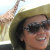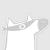International Conference on Automatic Face and Gesture Recognition是研究基于图像和视频的人脸、手势和身体运动识别的首要国际论坛。其广泛的范围包括：计算机视觉、模式识别和计算机图形学的基础进展；与面部、手势和身体运动相关的机器学习技术；新的算法和应用。官网链接：http://fg2019.org/0+0+0+0+0+0+0+0+0+0+0+0+0+0+0+0+0+0+0+0+0+0+0+0+0+0+0+0+0+0+0+0+0+0+0+0+0+0+0+0+0+0+0+0+0+
Top